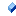The data members of the ExplodeParameters class are listed here.

 Name DescriptionThe force applied for each geometries generated by the explosion cis multiplied by a factor called Amplitude. The base force is computed according to the geometry mass and postion from the exploded geometry's center. The Amplitude is used to multiply this force.Subdivisions count, along the X axis, of the geometry to be exploded.Subdivisions count, along the Y axis, of the geometry to be exploded.Subdivisions count, along the Z axis, of the geometry to be exploded.